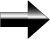Logarithms

Logarithms are very similar to exponents because they are based from the same principles as exponents.  They are, in effect, an inverse operation to exponents.

For example, if bx = N, then x = logb N.

Like exponents, there are only a few basic rules that you need to understand in order to master logarithms:

logb (PQ) = logb P + logb Q : This is the product rule for logarithms.  The reason that the logs add in this way is exactly the same reason why exponents with the same base add.

logb(P/Q) = logbP – logbQ:  As you can see, this is also exactly like exponents.  Remember, x-1 = 1/x, and so (P/Q) = P * Q-1, and so just like when dividing exponents with the same base, taking a log of

logb(P)r = r logb P:  This is because of the nature of logarithms.  It is essentially the same as the exponent operation (a2)3

So, Exponents and Logarithms are based of exactly the same principles.  If you can master one, then you can master both.  They are inverse operations of each other.

Main Algebra Page